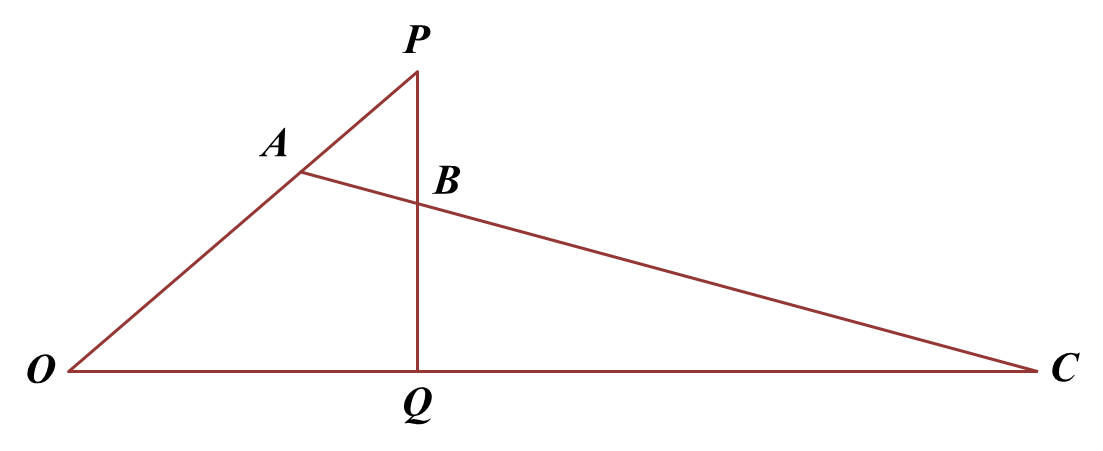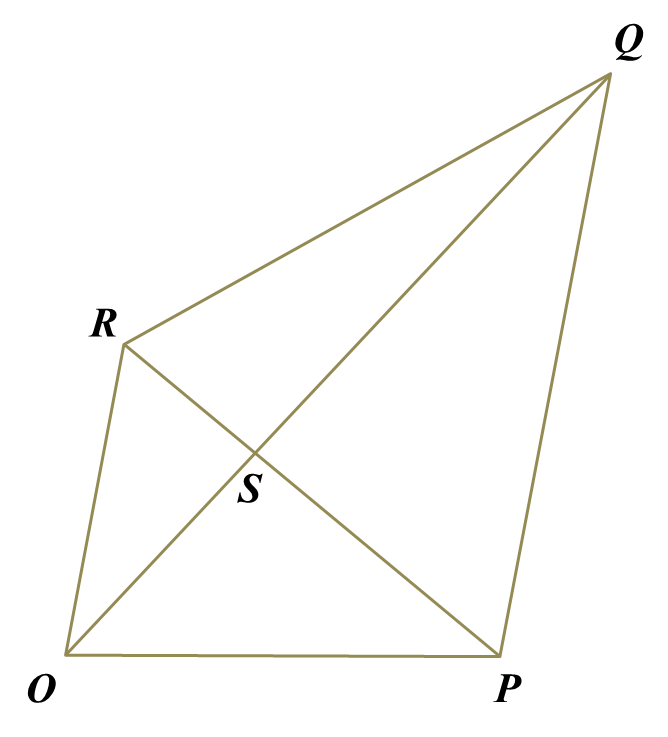# 4.7.4 Vectors, SPM Practice (Question 7 & 8)

Question 7:
Diagram below shows quadrilateral OPBC. The straight line AC intersects the straight line PQ at point B.Solution:
(a)

(b)

(c)(i)

(c)(ii)

Question 8:
Diagram below shows quadrilateral OPQR. The straight line PR intersects the straight line OQ at point S.Solution:
(a)(i)

(a)(ii)

(b)
$\begin{array}{l}\stackrel{\to }{PS}=\stackrel{\to }{PQ}-\stackrel{\to }{SQ}\\ \frac{3}{4}\stackrel{\to }{PR}=m\stackrel{\to }{OR}-n\stackrel{\to }{OS}\\ \frac{3}{4}\left(-7\underset{˜}{x}+5\underset{˜}{y}\right)=m\left(5\underset{˜}{y}\right)-n\left(\frac{7}{4}\underset{˜}{x}+\frac{15}{4}\underset{˜}{y}\right)\\ -\frac{21}{4}\underset{˜}{x}+\frac{15}{4}\underset{˜}{y}=5m\underset{˜}{y}-\frac{7}{4}n\underset{˜}{x}-\frac{15}{4}n\underset{˜}{y}\\ -\frac{21}{4}\underset{˜}{x}+\frac{15}{4}\underset{˜}{y}=-\frac{7}{4}n\underset{˜}{x}+5m\underset{˜}{y}-\frac{15}{4}n\underset{˜}{y}\\ -\frac{7}{4}n=-\frac{21}{4}\\ 7n=21\\ n=3\\ \\ 5m-\frac{15}{4}n=\frac{15}{4}\\ 5m-\frac{15}{4}\left(3\right)=\frac{15}{4}\\ 5m-\frac{45}{4}=\frac{15}{4}\\ 5m=15\\ m=3\end{array}$

(c)